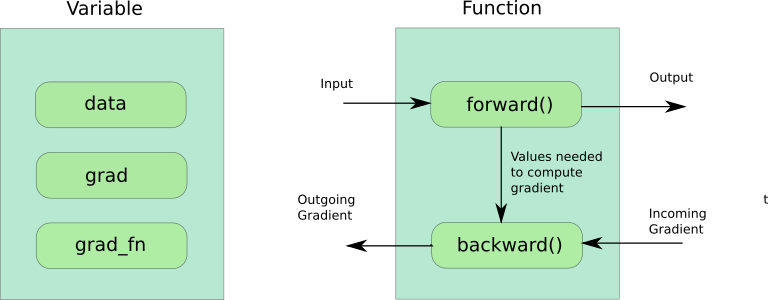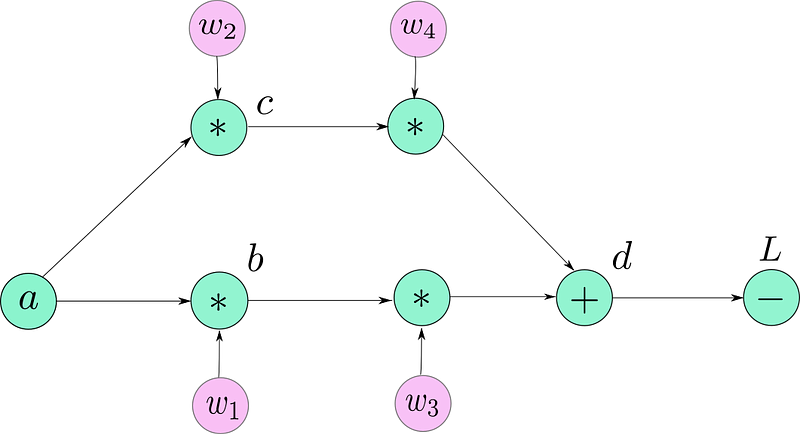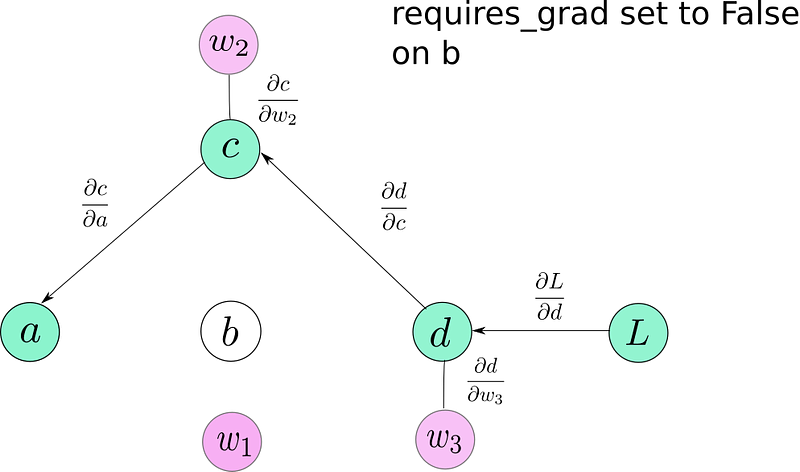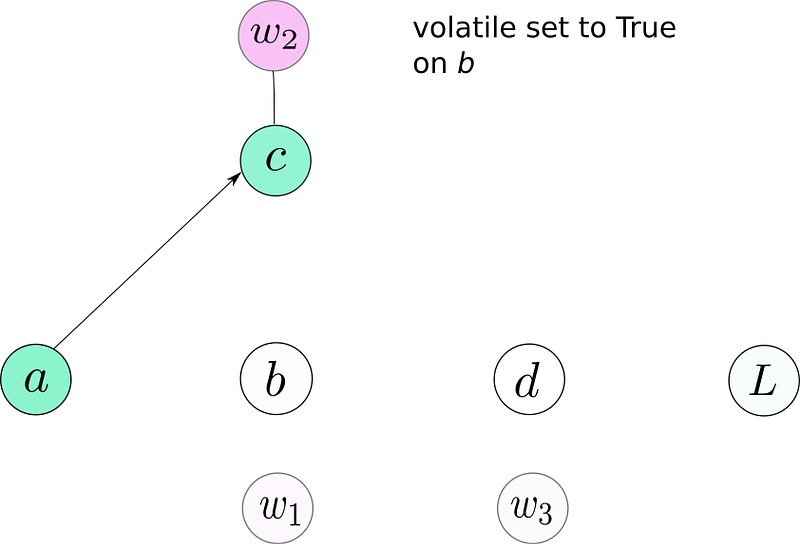# Getting Started with PyTorch Part 1: Understanding How Automatic Differentiation Works

PyTorch has emerged as a major contender in the race to be the king of deep learning frameworks. What makes it really luring is it’s dynamic computation graph paradigm.comments

### Building Block #3 : Variables and Autograd

PyTorch accomplishes what we described above using the Autograd package.

Now, there are basically three important things to understand about how Autograd works.

Building Block #3.1 : Variable

The Variable, just like a Tensor is a class that is used to hold data. It differs, however, in the way it’s meant to be used. Variables are specifically tailored to hold values which change during training of a neural network, i.e. the learnable paramaters of our network. Tensors on the other hand are used to store values that are not to be learned. For example, a Tensor maybe used to store the values of the loss generated by each example.

```from torch.autograd import Variable

var_ex = Variable(torch.randn((4,3))   #creating a Variable```

Variable class wraps a tensor. You can access this tensor by calling .dataattribute of a Variable.

The Variable also stores the gradient of a scalar quantity (say, loss) with respect to the parameter it holds. This gradient can be accessed by calling the .grad attribute. This is basically the gradient computed up to this particular node, and the gradient of the every subsequent node, can be computed by multiplying the edge weight with the gradient computed at the node just before it.

The third attribute a Variable holds is a grad_fn, a Function object which created the variable.NOTE: PyTorch 0.4 merges the Variable and Tensor class into one, and Tensor can be made into a “Variable” by a switch rather than instantiating a new object. But since, we’re doing v 0.3 in this tutorial, we’ll go ahead.

Building Block #3.2 : Function

Did I say Function above? It is basically an abstraction for, well, a function. Something that takes an input, and returns an output. For example, if we have two variables, and b, then if,

c = a + b

Then c is a new variable, and it’s grad_fn is something called AddBackward (PyTorch’s built-in function for adding two variables)the function which took a and b as input, and created c.

Then, you may ask, why is a need for an entire new class, when python does provide a way to define function?

While training neural networks, there are two steps: the forward pass, and the backward pass. Normally, if you were to implement it using python functions, you will have to define two functions. One, to compute the output during forward pass, and another, to compute the gradient to be propagated.

PyTorch abstracts the need to write two separate functions (for forward, and for backward pass), into two member of functions of a single class called torch.autograd.Function.

PyTorch combines Variables and Functions to create a computation graph.

Let us now dig into how PyTorch creates a computation graph. First, we define our variables.

The result of the above lines of code is,

Now, let’s dissect what the hell just happened here. If you look at the source code, here is how things go.

• Define the leaf variables of the graph (Lines 5–9). We start by defining a bunch of “variables” (Normal, python usage of language, not pytorch Variables). If you notice, the values we defined are the leaf nodes in the our computation graph. It only makes sense that we have to define them since these nodes aren’t result of any computation. At this point, these guys now occupy memory in our Python namespace. Means, they are hundred percent real. We must set the requires_grad attribute to True, otherwise, these Variables won’t be included in the computation graph, and no gradients would be computed for them (and other variables, that depend on these particular variables for gradient flow).
• Create the graph (Lines 12–15). Till now, there is nothing such as computation graph in our memory. Only the leaf nodes, but as soon as you write lines 12–15, a graph is being generated ON THE FLY. REALLY IMPORTANT TO NAIL THIS DETAIL. ON THE FLY. When you write b =w1*a, it’s when the graph creation kicks in, and continues until line 15. This is precisely the forward pass of our model, when the output is being calculated from inputs. The forward function of each variable may cache some input values to be used while computing the gradient on the backward pass. (For example, if our forward function computes W*x, then d(W*x)/d(W) is x, the input that needs to be cached)
• Now, the reason I told you the graph I drew earlier wasn’t exactly accurate? Because when PyTorch makes a graph, it’s not the Variable objects that are the nodes of the graph. It’s a Function object, precisely, the grad_fn of each Variable that forms the nodes of the graph. So, the PyTorch graph would look like.Each Function is a node in the PyTorch computation graph.

• I’ve represented the leaf nodes, by their names, but they too have their grad_fn’s (which return a None value . It makes sense, as you can’t backpropagate beyond leaf nodes). The rest of nodes are now replaced by their grad_fn’s. We see that the single node d is replaced by three Functions, two multiplications, and an addition, while loss, is replaced by a minus Function.
• Compute the Gradients (Line 18). We now compute the gradients by calling the .backward() function on L. What exactly is going on here? First, the gradient at L, is simply 1 (dL / dL). Then, we invoke it’s backwardfunction, which basically has a job of computing the gradients of the output of the Function object, w.r.t to the inputs of the Functionobject. Here, L is the result of 10 — d, which means, backwards function will compute the gradient (dL/dd) as -1.
• Now, this computed gradient is multiplied by the accumulated gradient (Stored in the grad attribute of the Variable corresponding to the current node, which is dL/dL = 1 in our case), and then sent to input node, to be stored in the grad attribute of the Variable corresponding to input node. Technically, what we have done is apply the chain rule (dL/dL) * (dL/dd) = dL/dd.
• Now, let us understand how gradient is propagated for the Variable d. d is calculated from it’s inputs (w3, w4, b, c). In our graph, it consists of 3 nodes, 2 multiplications and 1 addition.
• First, the function AddBackward (representing addition operation of node d in our graph) computes the gradient of it’s output (w3*b + w4*c) w.r.t it’s inputs (w3*b and w4*c ), which is (1 for both). Now, these localgradients are multiplied by accumulated gradient (dL/dd 1 = -1 for both), and the results are saved in the grad attribute of the respective input nodes.
• Then, the Function MulBackward (representing multiplication operation of w3*c) computes the gradient of it’s input output w.r.t to it’s inputs (w3 and c) as (c and w3) respectively. The local gradients are multiplied by accumulated gradient (dL/d(w3*c) = -1). The resultant value (-1 x c and -1 x w3) is then stored in grad attribute of Variables w3 and respectively.
• Gradients for all the nodes are computed in a similar fashion.
• The gradient of L w.r.t any node can be accessed by calling .grad on the Variable corresponding to that node, given it’s a leaf node (PyTorch’s default behavior doesn’t allow you to access gradients of non-leaf nodes. More on that in a while). Now that we have got our gradients, we can update our weights using SGD or whatever optimization algorithm you like.
`w1 = w1 — (learning_rate) * w1.grad    #update the wieghts using GD`

and so forth.

### Some Nifty Details of Autograd

So, didn’t I tell you you can’t access the grad attribute of non-leaf Variables. Yeah, that’s the default behavior. You can override it by calling .retain_grad()on the Variable just after defining it and then you’d be able to access it’s grad attribute. But really, what the heck is going on under the wraps.

Dynamic Computation Graphs

PyTorch creates something called a Dynamic Computation Graph, which means that the graph is generated on the fly. Until the forward function of a Variable is called, there exists no node for the Variable (it’s grad_fn) in the graph. The graph is created as a result of forward function of many Variables being invoked. Only then, the buffers are allocated for the graph and intermediate values (used for computing gradients later). When you call backward(), as the gradients are computed, these buffers are essentially freed, and the graph is destroyed. You can try calling backward() more than once on a graph, and you’ll see PyTorch will give you an error. This is because the graph gets destroyed the first time backward() is called and hence, there’s no graph to call backward upon the second time.

If you call forward again, an entirely new graph is generated. With new memory allocated to it.
By default, only the gradients (grad attribute) for leaf nodes are saved, and the gradients for non-leaf nodes are destroyed. But this behavior can be changed as described above.

This is in contrast to the Static Computation Graphs, used by TensorFlow where the graph is declared before running the program. The dynamic graph paradigm allows you to make changes to your network architecture duringruntime, as a graph is created only when a piece of code is run. This means a graph may be redefined during the lifetime for a program. This, however, is not possible with static graphs where graphs are created before running the program, and merely executed later. Dynamic graphs also make debugging way easier as the source of error is easily traceable.

This is an attribute of the Variable class. By default, it’s False. It comes handy when you have to freeze some layers, and stop them from updating parameters while training. You can simply set the requires_grad to False, and these Variables won’t be included in the computation graph. Thus, no gradient would be propagated to them, or to those layers which depend upon these layers for gradient flow. requires_gradwhen set to True is contagious, meaning even if one operand of an operation has requires_grad set to True, so will the result.is not included in the graph. No gradient is backpropagated through b now. a only gets gradients from now. Even if w1 has requires_grad = True, there is no way it can receive gradients.

volatile

This again is a attribute of a Variable class, which causes a Variable to be excluded from the computation graph when it is set to True. It might seem quite similar to requires_grad, given it’s also contagious when set True. But it has a higher precedence than requires_grad. A variable with requires_gradequals to True and volatile equals to True, would not be included in the computation graph.

You might think, what’s the need of having another switch to override requires_grad, when we can simply set requires_grad to False? Let me digress for a while.

Not creating a graph is extremely useful when we are doing inference, and don’t need gradients. First, overhead to create a computation graph is eliminated, and the speed is boosted. Second, if we create a graph, and since there is no backward being called afterwords, the buffers used to cache values are never freed and may lead to you running out of memory.

Generally, we have many layers in the a neural network, for which we might have set requires_grad to True while training. To prevent a graph from being made at inference, we can do either of two things. Set requires_grad False on all the layers (maybe, 152 of them?). Or, set volatile True only on the input, and we’re assured no resultant operation will result in a graph being made. Your choice.No graph is created for b or any node that depends on b.

NOTE: PyTorch 0.4 has no volatile argument for a combined Tensor/Variable class. Instead, the inference code should be put in a torch.no_grad() context manager.

```with torch.no_grad():
-----  your inference code goes here ----```

### Conclusion

So, that was Autograd for you. Understanding how Autograd works can save you a lot of headache when you’re stuck somewhere, or dealing with errors when you’re starting out. Thanks for reading so far. I intend to write more tutorials on PyTorch, dealing with how to use inbuilt functions to quickly create complex architectures (or, maybe not so quickly, but faster than coding block by block). So, stay tuned!

Bio: Ayoosh Kathuria is passionate about computer vision, and teaching machines how to extract meaningful information from their surroundings. He is currently working on improving object detection by leveraging context.

Original. Reposted with permission.

Related: